# IIT JEE Important Physics Formulas – JEE Main and Advanced

Preparation of JEE (Main and Advanced) is demanding as it is among the toughest competitive exams across the globe. One of the important phases of JEE preparation is to remember all the important formulas from the entire syllabus. There will be some questions where you can directly apply the formulas and get the answers.

Therefore, such questions will be very crucial in boosting your JEE scores. Here you will learn some useful ways of memorizing formulas related to JEE Physics section. There are around 47 chapters included in JEE Physics syllabus and remembering formulas from all these chapters is definitely a tough task. There are few simple ways of memorizing formulas included in JEE Syllabus:

1. Make a handmade note of all the formulas being highlighted. This would help in proper revision and a quick glance whenever required.

2. One of the most powerful tool, not only for remembering formulas but also for solving typical Physics questions in JEE Exams is dimensional analysis. While preparing you could do a comparative study between chapters like electrostatics and magnetostatics.

For example, the energy of electric dipole is given by U = – p.E. Similarly, the energy of a magnetic dipole is U = – μ .B C.

3. Additionally, remember to memorize the basic formulas like W = F.S and by using these formulas you can derive various other formulas like potential energy of a spring etc. You should memorize the values of different constants accurately because during the examination you might confuse between their values and that mistake could fetch you negative marking.

## IIT JEE Physics Important Concepts And Formulas

1. Electric Charge : Q = ± ne (e = 1.60218 × 10-29 C). The SI unit of Electric Charge is Coulomb (C)

2. Coulomb’s Law :

Electrostatic Force (F) = $\mathbf{k\;\frac{q_{1}\;q_{2}}{r^{2}}}$

And, in Vector Form : $\mathbf{\overrightarrow {F}\;=\;k\;\frac{\left ( q_{1}\;q_{2} \right )\; \times \;\overrightarrow {r}}{r^{3}}}$

Where,

q1 and q2 = Charges on the Particle,

r = Separation between them,

$\mathbf{\overrightarrow {r}}$ = Position Vector,

k = Constant = $\mathbf{\frac{1}{4\;\pi \;\epsilon _{0}}\;= \;8.98755\; \times \;10^{9}\;\;\frac{N\;m^{2}}{C^{2}}}$

3. Electric Current :

The current at Time t : $\mathbf{i\;=\;\lim_{\Delta t \rightarrow 0}\;\frac{\Delta Q}{\Delta t}\;=\;\frac{dQ}{dT}}$

Where,

Δ Q and Δ T = Charges crosses an Area in time Δ T

SI unit of Current is Ampere (A) and 1A = 1 C/s

4. Current Density: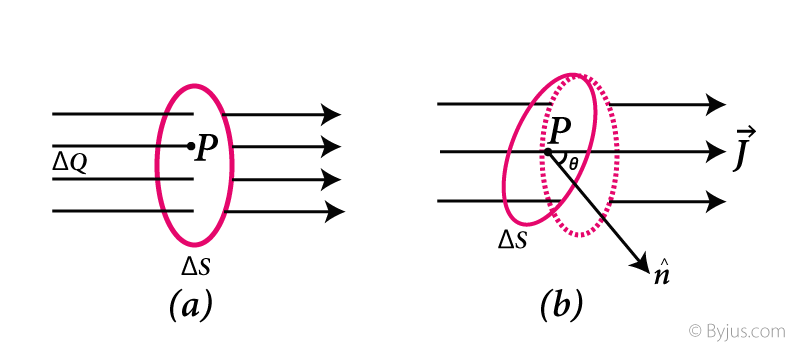Average current density :$\mathbf{\overrightarrow {j}\;=\;\frac{\Delta i}{\Delta s}}$

The Current Density at point P [Fig. a] : $\mathbf{j\;=\;\lim_{\Delta s \rightarrow 0}\;\frac{\Delta i}{\Delta s}=\frac{di}{dS}}$

The Current Density at point P [Fig. b] : $\mathbf{j\;=\;\frac{\Delta i}{\Delta S\;cos\; \theta }}$

Where,

Δ S = Small Area,

Δ i =Current through the Area Δ S,

P = Perpendicular to the flow of Charges,

θ = Angle Between the normal to the Area and the direction of the current.

5. Kirchrhoff’s Law: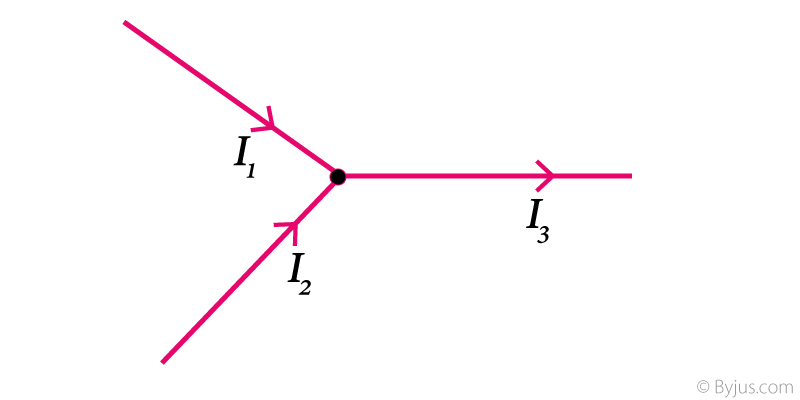Law of Conservation of Charge: I3 = I1 + I2

6. Voltage Law: The total voltage around a closed loop must be zero. $\mathbf{\sum \;V \;=\;0}$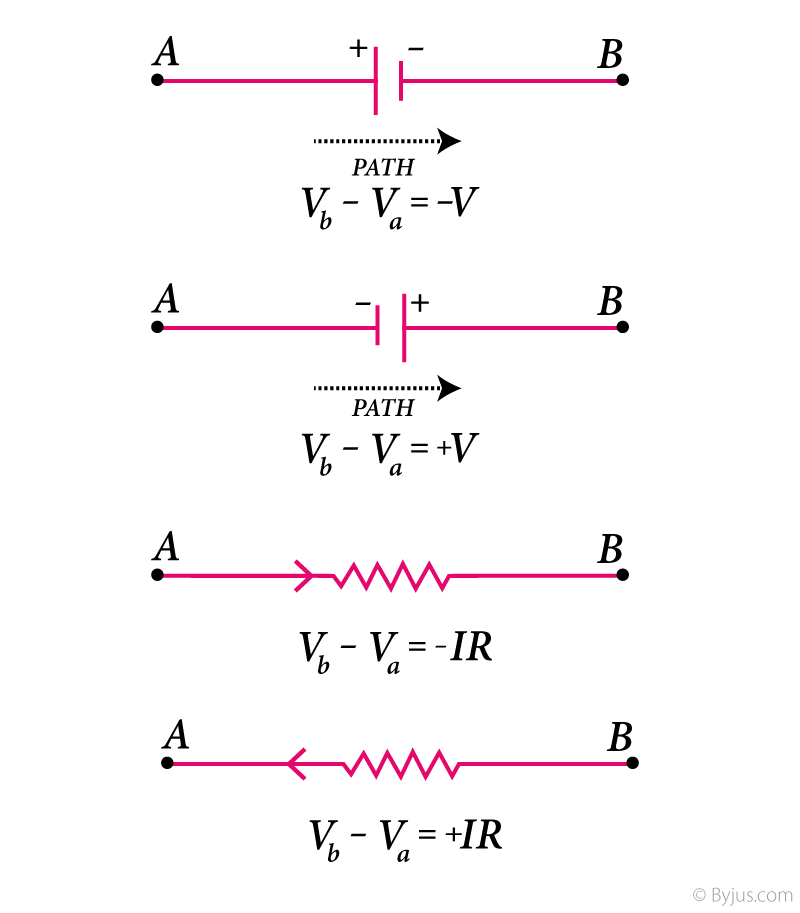Relationship Between Temperature and:

A. Resistivity : $\mathbf{ r\;\left ( T \right )\;=\; \rho \left ( T_{0} \right )\;\left [ 1\;+\;\alpha \left ( T\;-\;T_{0} \right ) \right ]}$

B. Resistance : $\mathbf{R \left ( T \right )\;=\;R \left ( T_{0} \right )\;\left [ 1\;+\;\alpha \left ( T\;-\;T_{0} \right ) \right ]}$

Where,

ρ(T) and ρ(T0) = Resistivity at Temperature T and T0 respectively,

α = Constant for given material.

7. Lorentz Force : $\mathbf{\overrightarrow {F}\;=\;q\;\left [ \overrightarrow {E}\;+\;\left ( \overrightarrow {v}\; \times \;\overrightarrow {B} \right ) \right ]}$

Where,

E = Electric Field,

B = Magnetic Field,

q = Charge of Particle,

v = Velocity of Particle.

8. Biot – Savart Law :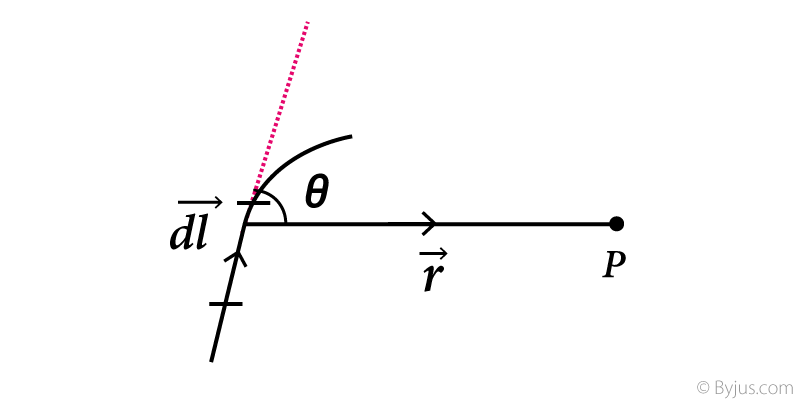Magnetic Field at Point P : $\mathbf{dB\;=\;\frac{mu _{0}}{4\;\pi }\;\frac{i\cdot dl\cdot Sin\; \theta }{r^{2}}}$

Where,

dl = Current Element and θ = Angle Between dl and r.

9. Magnetic Flux :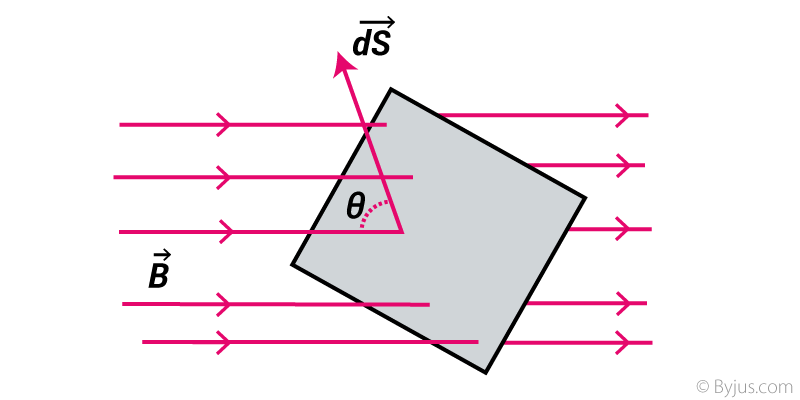Magnetic Flux through Area dS = $\mathbf{\phi \;=\; \overrightarrow {B}\;\cdot \;d\overrightarrow {S}\;=\;B\;\cdot \;dS\;Cos\; \theta }$

Where,

$\mathbf{d\overrightarrow {S}}$= Perpendicular vector to the surface and has magnitude equal to are Ds,

$\mathbf{\overrightarrow {B}}$ = Magnetic Field at an element,

θ = Angle Between $\mathbf{\overrightarrow {B}}$ and $\mathbf{d\overrightarrow {S}}$

SI unit of Magnetic Flux is Weber (Wb).

10. Equation of Motion:

A. Straight line Motion (Constant Acceleration):

1. $\mathbf{v\;=\;u\;+\;at}$

2. $\mathbf{s\;=\;ut\;+\;\frac{1}{2}\;at^{2}}$

3. $\mathbf{2as\;=\;v^{2}\;-\;u^{2}}$

B. Gravitational Acceleration:

 S.No Motion In Upward Direction Motion In Downward Direction A. V=u-gt V=u+gt B. $\mathbf{y\;=\;ut\;-\;\frac{1}{2}\;gt^{2}}$ $\mathbf{y\;=\;ut\;+\;\frac{1}{2}\;gt^{2}}$ C. $\mathbf{-\;2gy\;=\;v^{2}\;-\;u^{2}}$ $\mathbf{2gy\;=\;v^{2}\;-\;u^{2}}$

C. Projectile Motion:

horizontal Range (R) = $\mathbf{\frac{u^{2}\;sin\;2\; \theta }{g}}$

Time of Flight (T) = $\mathbf{\frac{2\;u\;Sin\; \theta }{g}}$

Maximum Height (H) = $\mathbf{\frac{u^{2}\;sin^{2}\; \theta }{2}}$

Where,

u = initial velocity,

v = final velocity,

a = constant acceleration,

t = time,

x = position of particle.

11. Universal Law of Gravitation: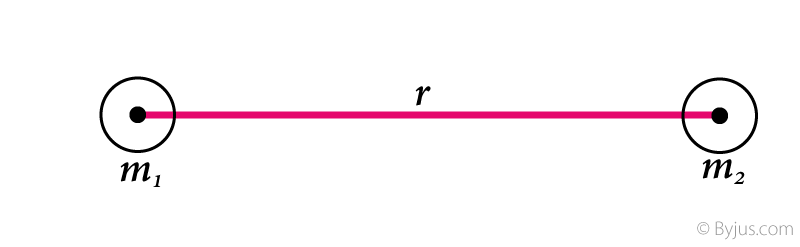Gravitational force $\mathbf{\overrightarrow {F}\;=\;G\;\frac{M\;m}{r^{2}}\;hat{r}}$

Where,

M and m = Mass of two Objects,

r = separation between the objects,

$\mathbf{\hat{r}}$ = unit vector joining two objects,

G = universal Gravitational Constant $\mathbf{\left [ \;G\;=\;6.67 \times 10^{-11}\;\;\frac{N \; \cdot \;m^{2}}{Kg^{2}} \; \right ]}$.

12. Work Done by Constant Force:Work Done (W) = $\mathbf{\overrightarrow {F}\;\cdot \;\overrightarrow {S}\;=\;\left | \overrightarrow {F} \right |\;\left | \overrightarrow {S} \right |\;cos\; \theta }$

Where,

S = Displacement along a straight line,

F = applied force,

θ = Angle between S & F.

It is a scalar quantity and the Dimension of work is [M1 L2 T-2]

SI unit of Work is joule (J) and $\mathbf{1\;J\;=\;1\;N\cdot m\;=\;\frac{Kg\;\cdot \;m^{2}}{s^{2}}}$

13. Centerof Mass: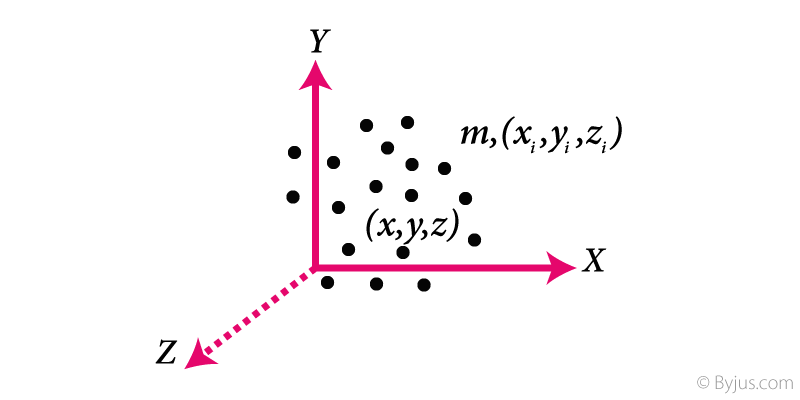The Position vector of Center of Mass : $\mathbf{\overrightarrow {R}_{CM}\;=\;\frac{1}{M}}\; \sum \;m_{i}\;\cdot \overrightarrow {r_{i}}$

Where,$\mathbf{\overrightarrow {r_{i}}}$ = position vector of ith particle.

The Coordinates of center of mass:

X = $\mathbf{\frac{1}{M}}\; \sum \;m_{i}\;\cdot \overrightarrow {x_{i}}$

Y = $\mathbf{\frac{1}{M}}\; \sum \;m_{i}\;\cdot \overrightarrow {y_{i}}$

Z = $\mathbf{\frac{1}{M}}\; \sum \;m_{i}\;\cdot \overrightarrow {z_{i}}$

Where,$\mathbf{\sum \;m_{i}}$ = Total Mass of the System (M)

14. Friction:

Kinetic Friction: fk = µk · N

Maximum Static Friction (limiting Friction): fmax = µs · N

Where, N = Normal Force,

µk = Coefficient of Kinetic Friction,

µs = Coefficient of Static Friction.

15. Lens Maker’s Formula:

General Equation for refraction (Spherical Surface): $\mathbf{\frac{mu _{2}}{v}\;-\;\frac{mu _{1}}{u}\;=\;\frac{mu _{2}\;-\;mu _{1}}{R}}$

Lens maker’s Formula: $\mathbf{\frac{1}{f}\;=\;\left ( mu \;-\;1 \right )\;\left ( \frac{1}{R_{1}}\;-\;\frac{1}{R_{2}} \right )}$

Lens Formula: $\mathbf{\frac{1}{f}\;=\;\frac{1}{v}\;-\;\frac{1}{u}}$

Where,

µ = Refractive Index,

u = Object Distance,

v = Image Distance,

f = focal length,

R1 and R2 = Radius of Curvature of the two side of the lens.

16. The Angle of Minimum Deviation: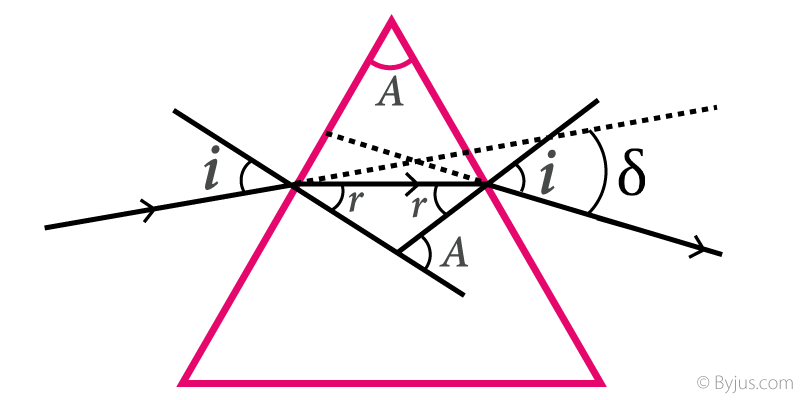$\mu\;=\;\frac{sin\left ( \frac{A\;+\;\Delta _{m}}{2} \right )}{sin \;\frac{A}{2}}$

Where,

µ = Refractive Index,

A = Internal Angle Of Prism,

$\mathbf{\Delta _{m}}$ = Angle of Minimum Deviation.

17. Simple Harmonic Motion:

Force (F) = – k x and k = ω2 m

Where,

k = Force Constant,

m = Mass of the Particle,

x = Displacement and ω2 = Positive Constant.

18. Simple Pendulum: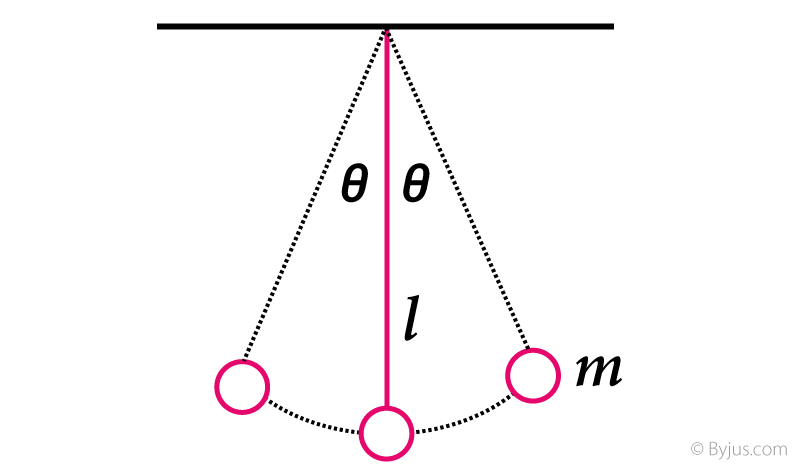Time Period (T) = $\mathbf{\frac{2\;\pi }{ \omega }\;=\;\sqrt{\frac{g}{l}}}$

Angular Frequency (ω) = $\mathbf{\sqrt{\frac{g}{l}}}$

Angular Acceleration (α) = $\mathbf{-\;\omega ^{2}\; \cdot \; \theta }$

Where, l = length of the String and m = Mass

19. Thermal Conductivity: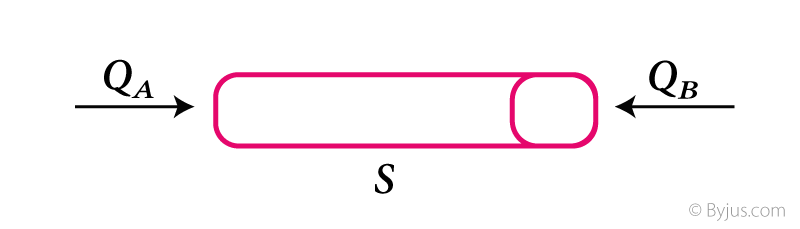Fourier’s Law : $\mathbf{\frac{\Delta Q}{\Delta T}\;=\;\frac{K\;A\;\left ( Q_{A}\;-\;Q_{B} \right )}{s}}$

Where,

K = Coefficient of Thermal Conductivity of Material,

QA = Temperature at the Left End,

QB = Temperature at the Right End,

Δ Q & Δ T = Amount of Heat Transfer (Q) through any Cross Section in time T,

s = Length of Solid Bar and A = Area of Cross section.

And, the SI Unit of Thermal Conductivity = $\mathbf{\frac{W}{m\;\cdot \;K}}$

Stay tuned with BYJU’S to know more about JEE Mains and JEE Advanced Examination Pattern, previous years question papers, sample papers, important questions and more.